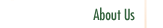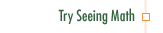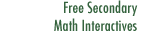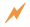Quadratic Functions

Participants in Quadratic Functions use models and problem solving to examine how the general nature of quadratic functions informs the particular instances described by quadratic equations. Participants also use multiple representations—tables, graphs, and equations—as powerful tools to describe physical situations.

Professional Development Goals

• Search for patterns and use quadratic functions to model physical situations
• Interpret the meaning and characteristics of quadratic functions as they appear in different representationsClick to Get Seeing Math™ SecondaryCourse Listing Proportional Reasoning Linear Functions Transformations of Linear Functions Linear Equations Systems of Linear Equations Quadratic Functions Transformations of Quadratic Functions Quadratic Equations Data Analysis